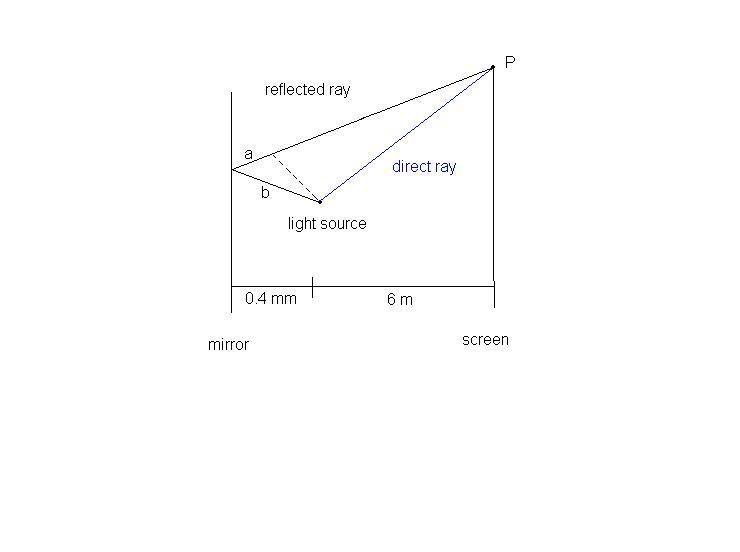# Interference question

I have run into a problem and I haven't been able to even begin figuring it out:

A point source of light with wavelength 589 nm is placed 0.4 mm above a mirror. Interference fringes are observed on a screen 6 m away, and the interface is between the light coming from the source and the light reflected off the mirror. Find the spacing of the fringes.

-I know that fringes will occur every time the phase changes by 2 pi, and that in this case the phase change of the reflected light will be:

(difference of path)(2 pi)/wavelength

but I'm not sure if reflecting off the mirror causes a pi phase change cause the index of refraction of the mirror is not given, so I can't tell if it is greater than the index of air. But my real problem is finding the path difference and relating it to the screen that is 6m away. Any hints on where to begin?

I just found that mirrors do cause a phase change of pi, but I still can't figure out how to relate path difference to the fringes on the screen

Hootenanny
Staff Emeritus
Gold Member
If we assume that the wave is reflected back along its line of propagation, then the phase angle between the reflected ray and the original ray will be $\pi$as you say. So now you have two sources of light with a phase angle of $\pi$ between them and a path difference of 0.4mm. Does that make sense?

Edit: So you figured the first bit out while I was typing (guess I should type faster). Now, dark fringes occur when the two waves incident on the screen have a phase angle of $n\pi$ where $n\in\mathbb{Z}$. Since your two wave sources now have a phase angle of $\pi$ destructive interference will occur when the path difference is an integer number of times the wavelength of the light. Do you follow?

Last edited:
I was under the impression their path difference would only be 0.4mm for the light ray reflecting off the mirror directly behind the light source.

Maybe this picture will help:Phase difference at point P will be:
pi + (a + b)(2 pi) / wavelength

Maybe I need to find a function that gives the phase shift at point P in terms of (a + b)...not sure though

andrevdh
Homework Helper
Put point P a distance 0.4 mm on the other side of the mirror (P) along the perpendicular to the surface of the mirror. A reflected ray will then travel straight from P to a point on the screen.